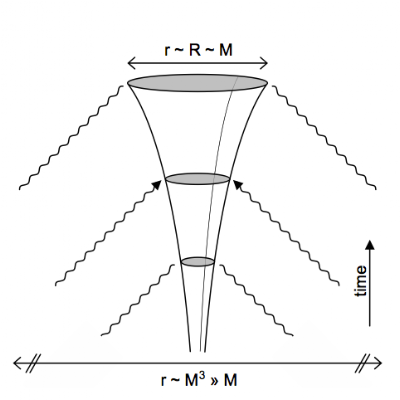## Saturday, August 07, 2010

### Entropy of black and white holes

Below are some remarks on black (and white) hole entropy which may help clarify the discussion (see also comments) elicited by Lubos Motl's blog post on my recent paper.

Apologies to most of my readers, who are not theoretical physicists! We will return to blogging on other topics soon :-) If you like this kind of discussion, see Lubos' blog -- he is one of the few people on the planet who can write with clarity and frequency on these topics!

In the discussion below I define entropy to be the logarithm of the number of distinct quantum states which are consistent with a particular coarse grained description of an object. For example, suppose there are N quantum states that correspond (when probed by a coarse detector) to a ball of stuff with ADM energy M and radius R. Then the entropy of this object is S = log N. Also note that I assume purely unitary evolution of quantum states.

In my paper I was interested in white holes described by the time reversal of a black hole spacetime. I was specifically interested in white holes in isolation -- i.e., surrounded by vacuum. If the black hole was formed by the collapse of some object (e.g., a star), the corresponding white hole will "explode" at some point, disgorging the original star. Lubos points out that this sequence of events is unlikely on entropic grounds: if one assumes that the white hole has entropy of order of order its area in Planck units, and the matter disgorged has much less entropy than this, the white hole explosion seems to violate the second law of thermodynamics. Do the white holes I consider actually have area entropy?

Note that there is a one to one mapping (via time reversal) of black hole and white hole interior states. The subset of black holes that are formed by the collapse of ordinary objects like stars has much less than area entropy. In fact, their entropy is bounded by a coefficient not much larger than unity times their area to the 3/4 power: A^{3/4}. This can be deduced from an entropy bound on ordinary matter in nearly flat space (see links below). Using the one to one property mentioned above, this gives us an estimate of the entropy of white holes which explode into ordinary matter like a star or dust ball.

How do we produce the black holes that account for the vast majority of the A entropy? Not by ordinary astrophysical processes. Instead, we have to start with a small black hole and allow it to slowly accrete energy. (There are other methods; see links below.) However, a black hole which slowly accretes energy will at the same time emit Hawking radiation. The time reversal of this kind of hole is not an isolated white hole, it is a white hole bathed in incoming radiation from the past light cone. This type of (most entropic) black hole IS quite similar to its time reversed white hole counterpart, unlike the isolated holes I studied. See figure below.I am making a distinction between two types of coarse grained objects: 1. black hole of mass M, but I know nothing more about the object or its history and 2. black hole of mass M, but I know it was formed from, e.g., a star. The two categories of objects have radically different entropy because in case 2 I can restrict the quantum state to a small subset of the states in case 1 (I have more information about the object).

To summarize, the white holes I studied are obtained via time reversal from a tiny subset all possible black holes. However, these are the black holes actually produced by known astrophysical processes (supernovae, galaxy formation, etc.), and their time reversed evolution does lead to an explosion. Whether such objects are of interest is left up to the reader :-)

Useful links:

Talk slides (source of the figure above).

A review article: Monsters, black holes and the statistical mechanics of gravity, http://arxiv.org/abs/0908.1265.

See also: What is the entropy of the universe? http://arxiv.org/abs/0801.1847, also discussed here (source of figure below).Figure 3 caption: Ordinary matter (star, galactic core, etc.) collapses to form an astrophysical black hole. Under unitary evolution, the number of final Hawking radiation states that are actually accessible from this collapse is $\sim \exp M^{3/2}$, i.e.~precisely the number of ordinary astrophysical precursors (\ref{th1}). It is therefore much smaller than the the number of $\sim \exp M^2$ states a black hole, and its eventual Hawking radiation, could possibly occupy if nothing about its formation process were known.